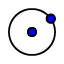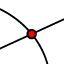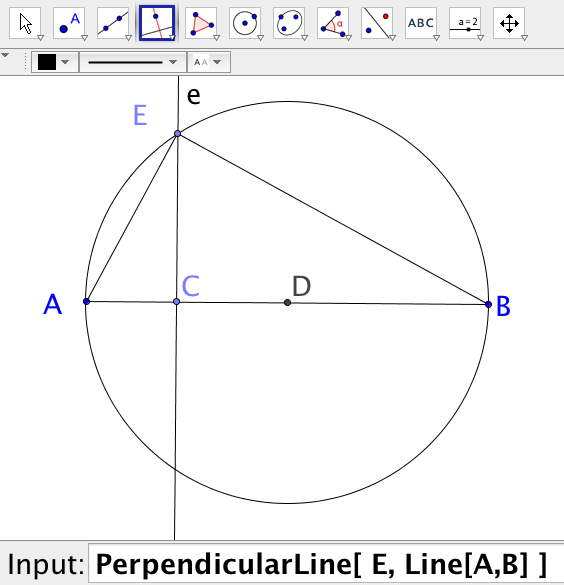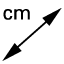# Geo-2017-Construction11-SimilarRightTriangles

## Objective:

Demonstrate & prove Einstein’s Lesser Theorem through measurement and proof.

## Visual Proof: Demonstration of Einstein's Lesser Thereom

How does the diagram above demonstrate that the blue and brown triangles are similar to the large triangle and to each other? (Hint: Consider the angles.)

## Constructing the Diagram:

1.Construct a circle of any diameter. 2.Construct a diameter using a line through the center and a point on the circumference. 3.Using the ends of the diameter andany other point on the circle, construct a triangle. 4.Construct the altitude of the triangle using the perpendicular line tool. 5.Label the intersection of the altitude and the base using the perpendicular line tool.

## Einstien's Lesser Theorem﻿Given a right triangle, an altitude drawn from the right angle to the hypotenuse will form two smaller triangles which are similar to each other and the original triangle.

## Observation & Measurement

Measure the following using the measurement toolor the Length command. CE by using Length[C,E] AC by using Length[A,C] CB by using Length[C,B] Calculate the product of and record it below. Calculate and record it on your diagram.

## Paragraph Proof

Given: EC is the altitude of the right Prove: